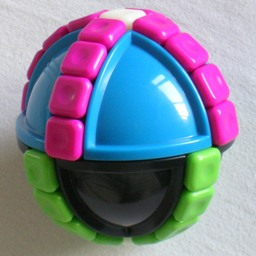# SphereXyzThe SphereXyz puzzle consists of two hemispheres, a black northern hemisphere and a blue southern one, that can twist with respect to each other. On the surface of this ball are two great circles, intersecting at the poles, consisting of small square tiles. Each ring has 18 tiles, making a total of 34 tiles since the two pole tiles are shared by both rings. In the solved position the pole tiles are white, the other 16 tiles in the black hemisphere are green and the remaining 16 tiles in the blue hemisphere are purple. A ring of tiles can be shifted as a whole, and by twisting the hemispheres sections of the rings are swapped.

This puzzle is closely related to the Massage Ball 2 puzzle, the main difference being that the SphereXyz does not have a ring around its equator.

This puzzle was invented and patented by Adam Giermek, WO 2008/030465, published 13 March 2010.

## The number of positions:

There are 34 tiles - 2 white, 16 green, and 16 purple. These can be rearranged in 34! / (2!·16!2) = 337,206,098,790 ways. All these positions can be achieved.

## Solution:

Phase 1: Pair up the white tiles.

1. Do whatever moves necessary to bring the two white tiles into different rings.
2. Rotate the rings so that one white tile is directly to the left of the North pole, and the other directly to the front of the South pole.
3. Rotate the Northern hemisphere clockwise, so that the two white tiles are now opposite each other on the sphere.

Phase 2: Pair up the green and purple tiles.

1. Find a green tile that has a green tile as its antipode on the opposite side of the sphere.
2. Find a purple tile that has a purple tile as its antipode on the opposite side of the sphere.
3. Using ring shifts only, bring the two pairs next to each other, and then slide them down to straddle the equator.
4. Hold the puzzle so that the pairs are at the front and back. So the tile just above the equator at the front is the same colour as the tile at the back just below the equator, and the tile just below the equator at the front is the same colour as the tile at the back just above the equator.
5. Look at the front tile just above the equator, and compare it to the tile just above that. If they have the same colour, then do the following move sequence:
1. Turn the top hemisphere 180 degrees.
2. Shift the front tiles upwards one tile.
3. Turn the top hemisphere 180 degrees.
4. Shift the front tiles downwards one tile.
5. Turn the top hemisphere 180 degrees.
6. Shift the front tiles downwards one tile.
7. Turn the top hemisphere 180 degrees.
8. Shift the front tiles upwards one tile.
If they have different colours, then instead to the inverse of that move sequence, namely:
1. Shift the front tiles downwards one tile.
2. Turn the top hemisphere 180 degrees.
3. Shift the front tiles upwards one tile.
4. Turn the top hemisphere 180 degrees.
5. Shift the front tiles upwards one tile.
6. Turn the top hemisphere 180 degrees.
7. Shift the front tiles downwards one tile.
8. Turn the top hemisphere 180 degrees.
6. Repeat a-e until every green tile has a purple antipode, and vice versa.

Phase 3: Solve the colours.
The puzzle can now be solved by shifting the rings only.

1. Shift the white tiles to the poles.
2. Examine the tiles in the front part of the ring. If there is any tile that has the wrong colour, then:
Shift that front tile upwards to the North pole.
Shift the other ring through 180 degrees.
Shift the front tiles down until the poles are white again.
3. Repeat step b until all the tiles at the front (and the back) have the right colour.
4. Rotate the whole puzzle a quarter turn so that the other ring comes to the front.
5. Repeat step b for this ring until it too is solved. The other ring will temporarily be upside down occasionally.
6. If the other ring has ended upside down, then turn it 180 degrees.